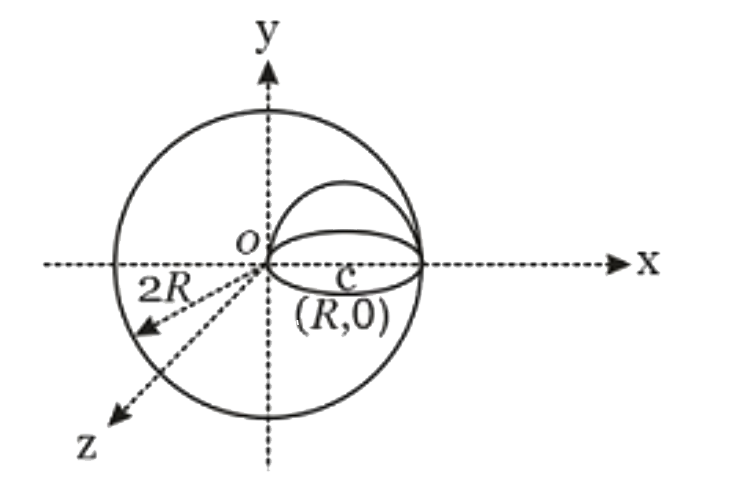# A hemispherical cavity of radius R is created in a solid sphere of radius 2R as shown in the figure . Then y -coordinate of the centre of mass of the remaining sphere isA

YCM=R15

B

YCM=R40

C

YCM=R30

D

YCM=R20

Updated on:21/07/2023
Text Solution
Verified by Experts